Courses

# Basics of Semiconductor Memories (Part - 2) Electrical Engineering (EE) Notes | EduRev

## VLSI System Design

Created by: Machine Experts

## Electrical Engineering (EE) : Basics of Semiconductor Memories (Part - 2) Electrical Engineering (EE) Notes | EduRev

The document Basics of Semiconductor Memories (Part - 2) Electrical Engineering (EE) Notes | EduRev is a part of the Electrical Engineering (EE) Course VLSI System Design.
All you need of Electrical Engineering (EE) at this link: Electrical Engineering (EE)

Objectives

In this lecture you will learn the following

• DRAM Basics
• Differential Operation In Dynamic RAMs
• DRAM Read Process With Dummy Cell
• Operation Of The Read Circuit
• Calculation Of Change In Bitline Voltage
• Area Considerations
• Metal Gate Diffusion Storage

29.1 DRAM Basics

A typical 1-bit DRAM cell is shown in Figure 29.11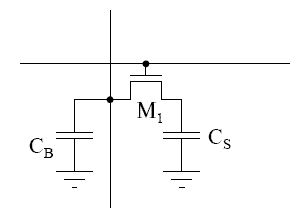Fig 29.11: DRAM Cell

The capacitor Cs stores the charge for the cell. Transistor M1 gives the R/W access to the cell. CB is the capacitance of the bit line per unit length.

Memory cells are etched onto a silicon wafer in an array of columns (bit lines) and rows (word lines). The intersection of a bit line and word line constitutes the address of the memory cell.

DRAM works by sending a charge through the appropriate column (CAS) to activate the transistor at each bit in the column. When writing, the row lines contain the state the capacitor should take on. When reading, the sense amplifier determines the level of charge in the capacitor. If it is more than 50%, it reads it as "1" ; otherwise it reads it as "0". The counter tracks the refresh sequence based on which rows have been accessed in what order. The length of time necessary to do all this is so short that it is expressed in nanoseconds (billionths of a second). e.g. a memory chip rating of 70ns means that it takes 70 nanoseconds to completely read and recharge each cell.

The capacitor in a dynamic RAM memory cell is like a leaky bucket. Dynamic RAM has to be dynamically refreshed all of the time or it forgets what it is holding. This refreshing takes time and slows down the memory.

29.2 Differential Operation In Dynamic RAMs

The sense amplifier responds to difference in signals appearing between the bit lines. It is capable of rejecting interference signals that are common to both lines, such as those caused by capacitive coupling from the word lines. For this common-mode to be effective, both sides of the amplifier must be matched, taking into account the circuit that feed each side. This is required in order to make the inherently single ended output of the DRAM cell appear differential.

Single To Differential Conversion:

Large memories (>1Mbit) that are exceedingly prone to noise disturbances resort to translating the single ended sensing problem into a differential one. The basic concept behind the single to differential is demonstrated in Figure 29.21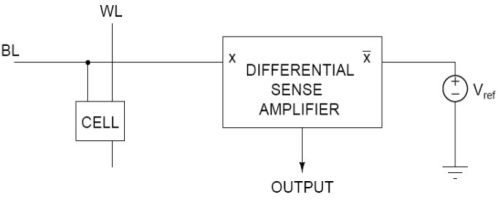Fig 29.21: Single to differential conversion

A differential sense amplifier is connected to a single ended bit line on one side and a reference voltage positioned between the "0" and "1" level at the other end. Depending on the value of BL the flip flop toggles in one or the other direction. Voltage levels tend to vary from die to die or even a single die so the reference source must track those variations. A popular way of doing so is illustrated in Figure 29.31 for the case of 1T DRAM. The memory array is divided into two halves, with the differential amplifier placed in the middle. On each side, a column called Dummy cell is added, these are 1T memory cells that are similar to the others, but whose sole purpose is to serve as reference. This approach is often called Open bit line archiecture.

29.3 DRAM Read Process With Dummy Cell

Circuit Construction:

The circuit is illustrated in Figure 29.31. Each bit line is split into two identical halves. Each half line is connected to half cells in the column and an additional cell known as Dummy cell having a capacitor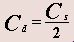When a word line on the left side is selected for reading the Dummy cell on the right side (controlled by XR) is also selected and vice versa, i.e. when a word line on the right side is selected the Dummy cell on the left (controlled by XL) is also selected. In effect, then, the Dummy cell operation serves as the other half of a differential DRAM cell. When the left bit line is in operation, the right half bit line acts as Complement for b line and vice versa. These cells shown here are the cells of a column, but look like a row. The distribution of the select lines are such that the even X's are in the right half and all the odds are in the left half.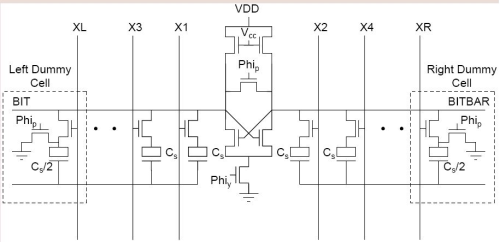Fig 29.31: Arrangement for obtaining differential operation from the single ended DRAM cell

29.4 Operation Of The Read Circuit

The circuit is shown in the previous slide in Figure 29.31. The two halves of the line are precharged to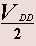and their voltages are equalized. At the same time, the capacitors of the two Dummy cells are precharged to. Then a word line is selected, and the Dummy cell of the other side is enabled (with and raised to VDD). Thus the half line connected to the selected cell will develop a voltage increment (above) of v or v0 depending on whether a "1" or "0" is stored in the cell. Meanwhile the other half of the line will have its voltage held equal to that of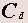(i.e.) the result is a differential signal that the sense amplifier detects and amplifies when it is enabled. As usual by the end of the regenerative process, the amplifier will cause the voltage on one half of the line to become VDD and that on the other half to become 0.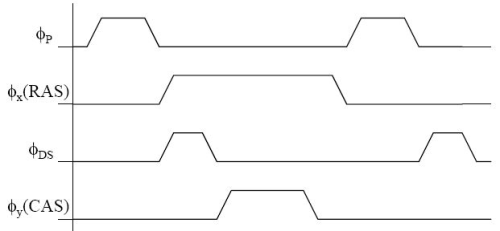Fig 29.41: Timing diagram of DRAM operation

If X1 cell is accessed, then the dummy cell on the right side is also selected.

Then the actual voltage of the bit line will be VBIT, where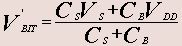where Cis BIT capacitance and Cis storage cell capacitance. Vs is voltage between the nodes of the storage capacitance. The storage capacitance with dummy cell is taken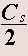on both sides in a column.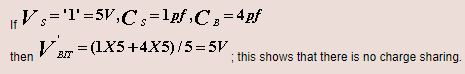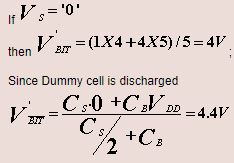(which is between 5 and 4)

The tricky part of a DRAM cell lie in the design of the circuitry to read out the stored value and the design of the capacitor to maximise the stored charge/minimise the storage capacitor size. Stored values in DRAM cells are read out using sense amplifiers, which are extremely sensitive comparators which compare the value stored in the DRAM cell with that of a reference cell. The reference cell used is a dummy cell which stores a voltage halfway between the two voltage levels used in the memory cell (experimental multilevel cells use slightly different technology). Improvements in sense amplifiers reduce sensitivity to noise and compensate for differences in threshold voltages among devices.

29.5 Calculation Of Change In Bitline Voltage

An array of DRAM cells is laid out as in Figure 29.21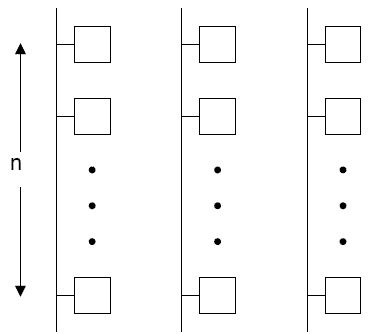Fig 29.21: Array of DRAM Cells

Let the capacitance per unit length of bitline be Cand the storage capacitance of the DRAM cell be CS. If there are n such sections (as shown) then the net bit capacitance of the bitline is nCs.

Let the bitline be precharged to Vp. So when the bitline is connected to the capacitance of the DRAM cell, the net voltage will be some intermediate voltage due to charge sharing and is given by: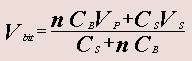(Eq 29.1)

A term Charge Transfer Ratio is defined in this context as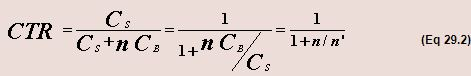where n' is defined as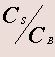For a particular technology, CB is fixed. So only Ct can be changed. When the bitline is connected to storage capacitor, the change of voltage at the bitline is given by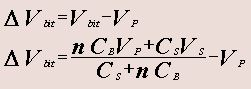(Eq 29.3)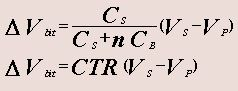For good design the value of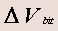(the change in voltage at bitline) should be as high as possible, so that it will allow the sensor to sense the bit correctly and quickly. Increase in Δ V requires CTR to increase. That leads to increase in the value of n'. n' depends on Cs and Cs. Thus to increase n' the storage capacitance Cs can be increased or the bitline capacitance CB can be decreased or both can be done. However increasing the value of storage capacitance requires larger area.

29.6 Area Considerations

To reduce the area requirement, still allowing the larger capacitance for storage, retrofit technique is used. In this case, the capacitors are laid down as shown below in Figure 29.31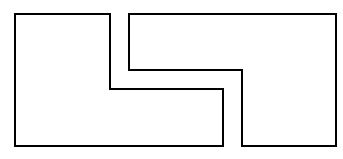Fig 29.31: Retrofit Technique

Standard DRAM cell uses diffusion, poly or metal as the bit line which will be discussed later.

Typical area calculation for storage capacitance is illustrated in Figure 29.32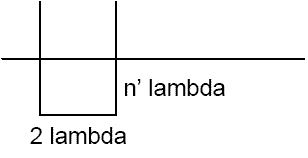Fig 29.32: Area Calculation

Area, A, consumed by the capacitor is given by: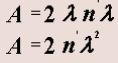(Eq 29.4)

Large area leads to large storage capacitance. Large storage capacitance leads to large change in bit voltageand therefore the access time will be small. DRAM cell with small access time can be designed by improvement on cell itself and the sense amplifier.

29.4 Metal Gate Diffusion Storage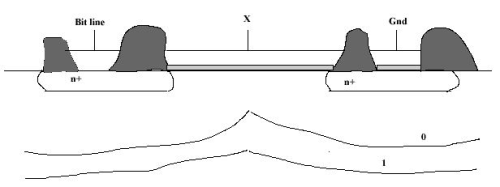Fig 29.41: Metal Gate Diffusion Storage

In this case the charge is stored in the depletion capacitance of the substrate and diffusion region. The problem with this design are:

• Diffusion line has higher capacitance. Thus CB increases and hence CTR decreases.
• Parasitic capacitances are higher and the gate is not self-aligned.
• There is routing problem associated with this kind of design.

A very much similar configuration can be used for inversion storage. In this case when gate input goes HIGH, the inversion charge stored in the capacitor is drained out and the potential at the channel drops indicating a '1' was stored. The reverse phenomena occurs if a '0' is stored.

Several DRAM cells are in place now. Few of them are as follows:

• SPDB - Single Poly Diffused Bit
• SPPB - Single Poly Poly Bit
• SPMB - Single Poly Metal Bit

Similarly it's possible to have:

• DPDB - Dual Poly Diffused Bit
• DPPM - Dual Poly Poly Bit
• DPMB - Dual Poly Metal Bit

Layout of a SPDB is shown in Figure 29.42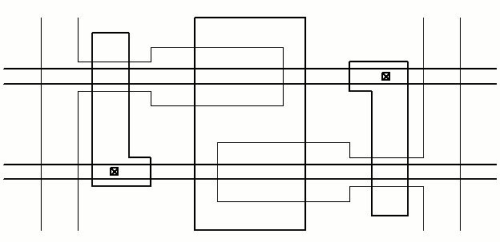Fig 29.42: Layout of SPDB Cell

Offer running on EduRev: Apply code STAYHOME200 to get INR 200 off on our premium plan EduRev Infinity!

35 docs|28 tests

,

,

,

,

,

,

,

,

,

,

,

,

,

,

,

,

,

,

,

,

,

;